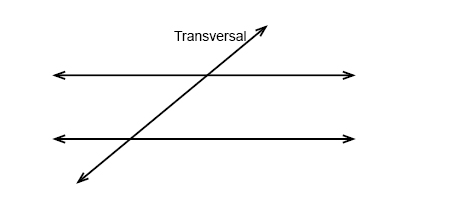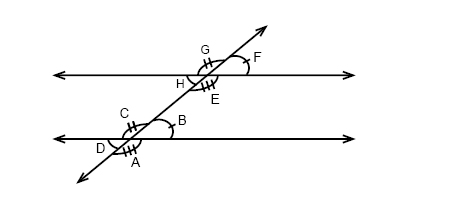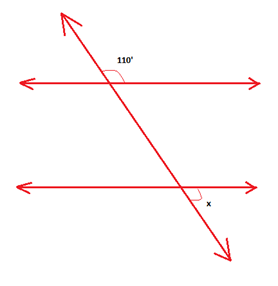# Angles, parallel lines and transversals

Two lines that are stretched into infinity and still never intersect are called coplanar lines and are said to be parallel lines. The symbol for "parallel to" is //.

If we have two lines (they don't have to be parallel) and have a third line that crosses them as in the figure below - the crossing line is called a transversal:In the following figure:If we draw to parallel lines and then draw a line transversal through them we will get eight different angles.

The eight angles will together form four pairs of corresponding angles. Angles F and B in the figure above constitutes one of the pairs. Corresponding angles are congruent if the two lines are parallel. All angles that have the same position with regards to the parallel lines and the transversal are corresponding pairs.

Angles that are in the area between the parallel lines like angle H and C above are called interior angles whereas the angles that are on the outside of the two parallel lines like D and G are called exterior angles.

Angles that are on the opposite sides of the transversal are called alternate angles e.g. H and B.

Angles that share the same vertex and have a common ray, like angles G and F or C and B in the figure above are called adjacent angles. As in this case where the adjacent angles are formed by two lines intersecting we will get two pairs of adjacent angles (G + F and H + E) that are both supplementary.

Two angles that are opposite each other as D and B in the figure above are called vertical angles. Vertical angles are always congruent.

$\angle A\; \angle F\; \angle G\; \angle D\;are\; exterior\; angles\\ \angle B\; \angle E\; \angle H\; \angle C\;are\; interior\; angles\\ \angle B\;and\; \angle E,\; \angle H\;and\; \angle C\;are\; consecutive\; interior\; angles\\ \angle A\;and\; \angle G,\; \angle F\;and\; \angle D\;are\; alternate\; exterior\; angles\\ \angle E\;and\; \angle C,\; \angle H\;and\; \angle B\;are\; alternate\;interior\; angles\\ \left.\begin{matrix} \angle A\;and\; \angle E,\; \angle C\;and\; \angle G\\ \angle D\;and\; \angle H,\; \angle F\;and\; \angle B\\ \end{matrix}\right\} \;are\; corresponding\; angles$

Two lines are perpendicular if they intersect in a right angle. The axes of a coordinate plane is an example of two perpendicular lines.

In algebra 2 we have learnt how to find the slope of a line. Two parallel lines have always the same slope and two lines are perpendicular if the product of their slope is -1.

## Video lesson

Find the value of x in the following figure